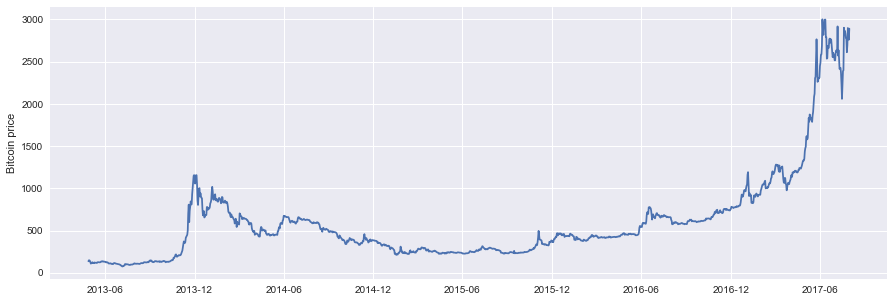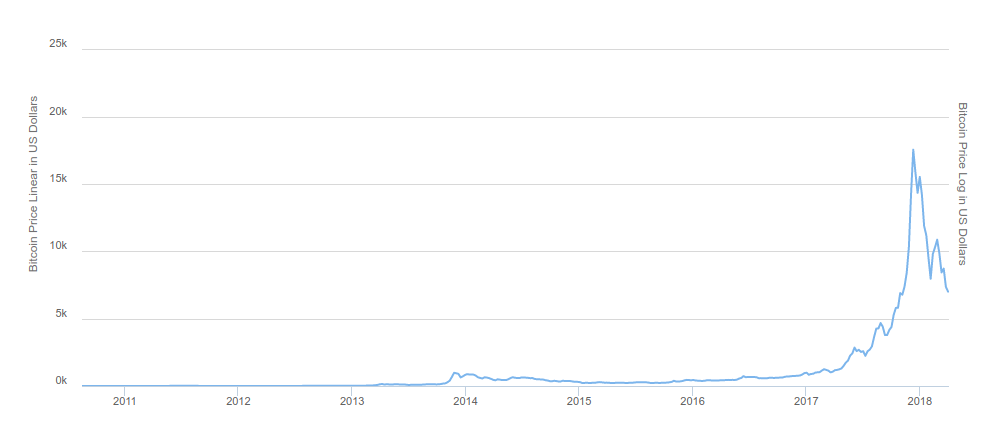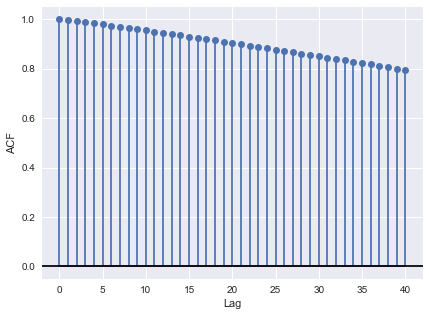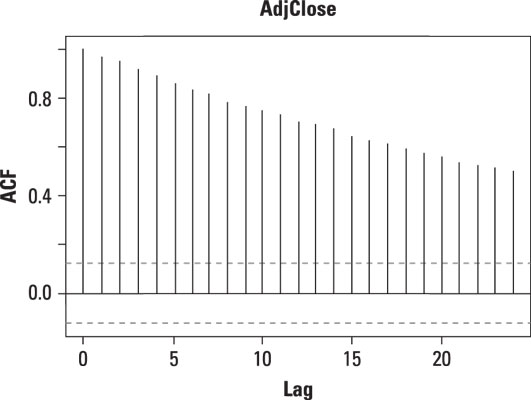# Blog

## Understanding Time Series Forecasting with Python

Vinta is a software studio whose focus is to produce high quality software and give clients great consulting advices to make their businesses grow. However, even though our main focus is web development, we also do our share of machine learning over here.

This article is the first of a few designed to show everything (or almost everything) you need to know about time series. It discusses what they are, how deal with them, how to choose forecast models and apply them to a real problem.

Time Series

Let’s start with time series, they are everywhere. From the total amount of rain that pours into a river per year, to stock markets, to weekly company sales, to speech recognition. But what are they?

Time series are:
"An ordered sequence of values of a variable at equally spaced time intervals."

Analyzing this ordered data can reveal things that at first where not clear, such as unexpected trends, correlations and forecast trends in the future bringing a competitive advantage to anyone who uses it. For these reasons it can be applied to a wide range of fields.

A forecasting task usually involves five basic steps.

1. Problem definition.
2. Gathering information.
3. Preliminary (exploratory) analysis.
4. Choosing and fitting models.
5. Using and evaluating a forecasting model.

We will go through all of them while analyzing bitcoin’s price.

#### 1. Problem definition.

In this post the problem to be exploited will be: what’s gonna be bitcoin’s price in the near future. How to forecast a high-risk asset, whose price can unpredictably increase or decrease over a short period of time, and that can also be influenced by a wide range of factors?

You may think this is an impossible mission, but forecasts rarely assume that the environment is not changing. What is normally assumed is that the way in which the environment is changing will continue in the future. That is, a highly volatile environment will continue to be highly volatile, a business with fluctuating sales will continue to have fluctuating sales, and an economy that has gone through booms and busts will continue that way. Of course this is not a magic box. Time series only uses information on the variable to be forecast, and makes no attempt to discover the factors which affect its behavior. Thus, it will extrapolate trend and seasonal patterns, but it ignores all other information, such as marketing initiatives, competitor activity, changes in economic conditions, and so on. Unless that the data and series are modeled for it, supposing that some of these things can be modeled, such as competitor activity. Therefore beware that will be limitations.

#### 2. Gathering information.

Building a dataset can be difficult and exhausting, which is why we’re going to use a Kaggle dataset. The goal is to build a model from the market data. This is a small dataset of bitcoin’s most important rates of the day. This will allow us to both train the models and see the results faster. Here’s a sample of this dataset:Kaggle dataset

We already know what to forecast and now we have the data. However, there are still a few things missing, like what's gonna be our forecast horizon - how far in the future we want to predict. One hour in advance, six months, ten years? Different types of models will be necessary, depending on what forecast horizon is most important. In this case, our forecast horizon will be one day, because the kaggle dataset contains the historical daily variation of the price.

#### 3. Preliminary (exploratory) analysis.

Step 3 is all about knowing the data. Is not the time to choose or build any model, it’s time to explore the dataset. Are there consistent patterns? Is there a significant trend? Is seasonality important? Is there evidence of the presence of business cycles? Are there any outliers in the data that need to be explained by those with expert knowledge? How strong are the relationships among the variables available for analysis? The answers to these questions will be valuable when choosing the forecast models.

To explore the data, we must see the data. Graphs allow us to visualize many features of the data, including patterns, unusual observations, changes over time, and relationships between variables.

To begin working with the data, start up a Jupyter Notebook. To plot the observations against the time of observation, load the data and use the dates as an index. After loading and indexing the data, it’s time to plot the graph. There are many python libraries like Pandas, and Matplotlib, that can assist in this process:

```import numpy as np
import pandas as pd
import matplotlib.pyplot as plt
import seaborn as sns; sns.set()
import pyflux as pf
from datetime import datetime
from dateutil.parser import parse
from datetime import datetime

%matplotlib inline

def convert(date):
holder = []
for i in date:
tp = parse(i).timestamp()
dt = datetime.fromtimestamp(tp)
holder.append(dt)
return np.array(holder)

data_location = '/btc/bitcoin_price_Training - bitcoin_price2013Apr-2017Aug.csv'

btc_data = btc_data[::-1]
date = btc_data['Date'].values
date_n = convert(date)
btc_data['Date'] = date_n
btc_data = btc_data.set_index('Date')

plt.figure(figsize=(15,5))
plt.plot(btc_data.index, btc_data['High'])
plt.ylabel('Bitcoin price')
```Jupyter notebook screenshot: Time Plots

After plotting the data, the next step would be data transformation. However, since we are using the Kaggle dataset, all transformations have already been made. We don’t have to worry about missing data or data transformation, which allows us to skip directly to using the data. Yet, if you are using another database or a built-in database, it’s imperative to transform the data before using it. In case you are using your own database, in this part, you should make sure that there is no data missing, and all the data is on the same format if you are working with text remove punctuation. The goal is to make sure your data is ready to be passed to your forecast models.

Analyzing the graph, some distinguishable patterns appear when we plot the data.

• The time-series has an overall increasing trend.
• At some point of 2014, the price passed the \$1,000 mark.
• After the 2014 peak, the price wouldn’t break the \$1,000 mark again for another three years.
• At some point of 2017, the price increases again.

This dataset is now outdated, the situation has changed a lot since them. The price continued to grow until the end of 2017 and then shrunk in half at the beginning of the year.Updated chart of bitcoin’s price

#### Time Series Decomposition

Time series data can exhibit a huge variety of patterns and it’s helpful to split a time series into several components, each representing one of the underlying categories of a pattern. Usually a time series can be segmented into four patterns.

• Trend: A trend exists when there is a long-term increase or decrease in the data. It does not have to be linear. Sometimes we will refer to a trend “changing direction” when it might go from an increasing trend to a decreasing trend.

• Seasonal: A seasonal pattern exists when a series is influenced by seasonal factors (e.g., the quarter of the year, the month, or day of the week). Seasonality always has a fixed and known period.

• Cycles: A cyclic pattern exists when data exhibit rises and falls that are not from the fixed period. The duration of these fluctuations is usually of at least 2 years.

• Noise: The random variation in the series.

To visualize these patterns, there is a method called ‘time-series decomposition’. As the name suggests, it allows us to decompose our time series into three distinct components: trend, seasonality, and noise. Statsmodels provides the convenient `seasonal_decompose` function to perform seasonal decomposition out of the box.

```import statsmodels.api as sm

# Beware seasonal_decompose() expects a DateTimeIndex on your DataFrame.
fig = decomposition.plot()
plt.figure(figsize=(15,5))
plt.show()
```

The result can be seen below:Jupyter notebook screenshot: Seasonal decomposition

• The seasonal part of the graph shows strong seasonality within each day.
• On the trend part of the graph, there is no seasonality, but an obvious rising trend.
• The graphs shows no evidence of any cyclic behaviour.
• The residual graph shows no trend, seasonality or cyclic behaviour. There are random fluctuations which do not appear to be very predictable.

Using time-series decomposition makes it easier to quickly identify a changing mean or variation in the data. These can be used to understand the structure of our time-series. The intuition behind time-series decomposition is important, as many forecasting methods build upon this concept of structured decomposition to produce forecasts.

#### Autocorrelation Function

Another way to know more about your time series is measuring the autocorrelation. The correlation between two functions (or time series) is a measure of how similarly they behave. Autocorrelation is a correlation coefficient. However, instead of correlation between two different variables, the correlation is between two values of the same variable at different times. This concept fits perfectly with one of the technical analysis’ main assumptions: history tends to repeat itself. And if it does, we wanna know how much it repeats.

We are going to use the autocorrelation function for the following purposes: Detect non-randomness in data. Identify an appropriate time series model if the data is not random.

The plot is also known as a correlogram.

```from statsmodels.tsa.stattools import acf
import matplotlib.pylab as plt

data = np.log(btc_data['High'])
lac_acf = acf(data, nlags=40)

plt.figure(figsize=(15,5))
plt.subplot(121)
plt.stem(lac_acf)
plt.axhline(y=0, linestyle='-', color='black')
plt.xlabel('Lag')
plt.ylabel('ACF')
plt.show()
```Jupyter notebook screenshot: ACF

• All correlograms start at 1; this is because when t=0, we are comparing the time series with itself.
• We can see that the time series is not random, but rather has a high degree of autocorrelation between adjacent and near-adjacent observations.
• This is a very similar graph to the Apple stock from January 1, 2013 to December 31, 2013.Autocorrelation plot of daily prices of Apple stock.

We now know a lot about time series, about they behavior. On the next post, we will go through the steps 4 (Choosing and fitting models) and 5 (Using and evaluating a forecasting model). We’ll discuss the tradeoff between statistical models and neural network-based techniques and how they perform.